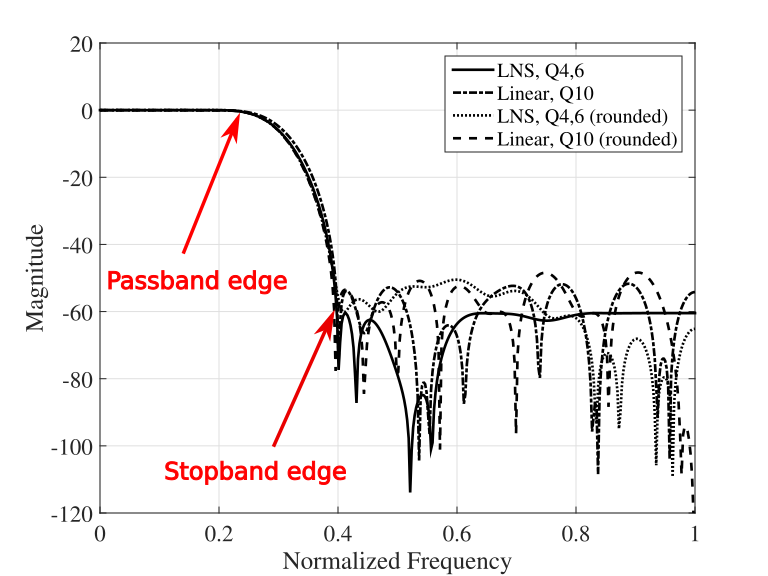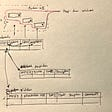# The Multiprocessing Library

Python provides a multiprocessing library where multiple processes can be spawned by the script and synchronized at some point. Variables can be shared between multiple processes including the parent process (the script that implements multiprocessing.

This article is not intended to be a comprehensive guide to use the multiprocessing library but just how I have used it for my limited use case. For complete details about the library refer to the page here:

## Use Case

My use case was running an optimizer for FIR filter. The optimizer implemented was finding solutions to an optimization problem. The optimization problem was minimizing the approximation error between the designed and ideal filter while designing an FIR filter. There were two cases, one designing the filter for integer filter coefficients and the other was for FIR filter coefficients in the log space (LNS), specifically log to the base-2.

The optimizer was to be run for the various combinations of the following parameters

• Filter Pass-band (ωcT) and stop-band edge (ωsT)
• Filter order (N)
• Number of integer and fractional bits for the LNS optimizer (total integer word length for the integer word length where the total integer word length is the sum of the integer and fractional word length)
• Base of LNS, ranging from √2 to 4.0 for the LNS optimizer

The pass-band and stop-band edge are shown here graphically for a low-pass FIR filter:Magnitude response for an FIR filter designed in the integer and LNS space. Image taken from “Syed Asad Alam, Oscar Gustafsson, “Design of Finite Word Length Linear-Phase FIR Filters in the Logarithmic Number System Domain”, VLSI Design, vol. 2014, Article ID 217495, 14 pages, 2014.”

So, the two optimizers were to be run for the combination of the above parameters with no correlation between the each run, bar one. Each optimizer run returned the approximation error which were to be written to an excel file for logging purposes. Thus, there needed to be a mechanism to track the approximation error returned by each run of the optimizer for logging purposes.

This scenario provided a perfect opportunity to make use of 12 cores in my workstation. Although in the end I was not successful in actually achieving it but the primary reason was the badly written script (I wrote it 8–9 years ago in Python2 with lots of global variables) for the LNS optimizer which hindered running it as a standalone function and returning some useful information. But in the attempt to do so, I was introduced to the `multiprocessing` library from Python.

## The `Process` Class

At the heart of the library is the `Process` class which can be used to initiate multiple processes. For example, the following code segment shows the creation of the process, the starting of the process and the point where one waits for the process to finish. A target function needs to be defined which will executed as a process with its arguments. The `p.start()` and `p.join()` are similar to the `fork` and `join` concept typically used in other languages supporting multiprocessing.

`from multiprocessing import Processdef func(name):    print(f'Hello {name}')def main():    p = Process(target=func, args=('Asad',))    p.start()    p.join()    return 0if __name__ == '__main__':    main()    exit(0)`

The output of the above code snippet is as follows:

`Hello Asad`

## Function with Return Argument — Using Pool Class

If the function returns an argument, there can be two ways to handle that. An example taken from the library documentation (but modified), one can use the Pool class which not only allows to run the same function across multiple input values while also handling the return values, as follows:

`from multiprocessing import Pooldef func(x):    return x*x*xdef main():    with Pool(5) as proc:        print(proc.map(func, [3, 5, 7]))    return 0if __name__ == '__main__':    main()    exit(0)`

This code snippet produces the following output:

`[27, 125, 343]`

## Function with Return Argument — Using Manager Class and Shared Variables

However, function can also return values through shared variables. These variables are provided by the Manager class. One can either use a single `Value` and or an `Array`. The `Value` variable needs to be initialized and passed to the target function where it can be used as a return variable without actually returning anything. The following code shows a small demo of how to test the `Value` variable:

`import multiprocessing as mpdef func(x1,x2,y):    y.value = x1+x2def main():    manager = mp.Manager()    return_value = manager.Value("i",0)    x1,x2 = 2,3    proc = mp.Process(target=func, args=(x1,x2,return_value))    proc.start()    proc.join()    print('##################################')    print('Demonstrating use of manager.Value')    print('##################################')    print(f'Return value: {return_value.value}')return 0if __name__ == "__main__":    main()    exit(0)`

The output produced by the following code snippet is:

`##################################Demonstrating use of manager.Value##################################Return value: 5`

The other way is to use the `Array` variable. This can be specially useful if the order in which each function returns a value is important. For example, in my use case of FIR filter, the approximation error returned by each optimizer run had to be logged against the changing word length for each base and each base against a filter order and eventually for multiple filter orders for each filter specification in terms of the band edges.

So the processes were spawned through a for loop which goes through the above defined parameters in order but each optimization run was running independently and finished out-of-order. Instead of producing the complicated code for the FIR optimizer, a minimal example can help explain the use of the `Array` variable better.

In the following example, a function adds two numbers and the result is saved in a shared `Array`. The index to where in the `Array` the result will be saved is also passed to the function. This index is important to make sure that each function call returns the result in the correct place in the `Array`. The function definition looks like this:

`def func(x1, x2, proc_num, return_array):    print(f'Process: {proc_num}')    return_array[proc_num] = x1+x2`

Each function is then spawned as a process and allowed to run independently. Each process is also made part of a list `jobs`. This list is then used as a synchronizing point so that one moves forward to process the `Array` only after all processes have finished execution. Of course one can do useful things before the processes finish work but they need to be independent of the parallel processes. The full code listing is:

`import multiprocessing as mpimport numpy as npdef func(x1, x2, proc_num, return_array):    print(f'Process: {proc_num}')    return_array[proc_num] = x1+x2def main():    print('##################################')    print('Demonstrating use of manager.Array')    print('##################################')    ### Multiprocessor Manager    manager = mp.Manager()    ### List of all processes    jobs = []    ### The shared Array object    return_array = manager.Array('i', np.arange(5))    ### Spawning the processes    for i in np.arange(5):        proc = mp.Process(target=func, args = (i,i*2,i,return_array))        ### Appending the processes to a list        jobs.append(proc)        ### Starting the process        proc.start()### Synchronizing all the processes    for proc in jobs:        proc.join()### Printing the shared array in various ways    print(f'Return array: {return_array}')    array_int = np.array(return_array)    print(f'Numpy array: {array_int}')    array_list = []    for i in range(len(return_array)):        array_list.append(return_array[i])    print(f'List: {array_list}')if __name__ == '__main__':    main()    exit(0)`

A key thing shown in the above code segment is that the array can be cast into other forms. Casting to a `numpy` array and a `list` is shown in this example. Individual elements of the array can also be converted to floating point numbers or integers, as per the requirements.

The output of the above code snippet is:

`##################################Demonstrating use of manager.Array##################################Process: 0Process: 2Process: 3Process: 1Process: 4Return array: array('i', [0, 3, 6, 9, 12])Numpy array: [ 0  3  6  9 12]List: [0, 3, 6, 9, 12]`

Even if one introduces calls a time.sleep() object in the function with random sleep times to illustrate that processes are being executed in parallel, use of an index variable can ensure that the `Array` is populated in the correct order, as shown here:

`##################################Demonstrating use of manager.Array##################################Process: 3Process: 0Process: 1Process: 2Process: 4Return array: array('i', [0, 3, 6, 9, 12])Numpy array: [ 0  3  6  9 12]List: [0, 3, 6, 9, 12]`

## Executing A Python Script from Another Script

To diverge a little from the topic, if one wants to run a python script from another script, one can use the `subprocess` module. From within the module, one can either use `Popen` or `call` Class. After `import subprocess`, to use the `Popen` class, pass the full command for python as a string. It is important to set the `shell` variable to True to enable shell execution, else it will not execute.

`cmd = 'python test.py --arg1 ' + str(arg1) + ' arg2 ' + str(arg2)s = subprocess.Popen(cmd, shell=True) # executed as a shell scripts.communicate() ### Now waiting for subprocess to complete`

The `s.communicate()` is to make the script wait for the `subprocess` to complete else the process is run in the background and the script moves forward. The `call` class does a blocking execution, removing the requirement to use the `s.communicate()` method. It can be used as follows:

`cmd = 'python test.py --arg1 ' + str(arg1) + ' arg2 ' + str(arg2)s = subprocess.call(cmd, shell=True) # executed as a shell script`

If a C/C++ executable file is to be run from a python script, there is no need to set the `shell` variable to `True`. Also, command line arguments can be passed in a different way:

`s = subprocess.Popen(['./test_cpp',str(arg1),str(arg2)])s.communicate() # Now waiting for subprocess to complete### Or use subprocess.calls = subprocess.call(['./test_cpp', str(arg1), str(arg2)]`

# Final Remarks

The code snippets shown above are not meant to be a complete and exhaustive tutorial but only what I learned and wanted to share with the wider community.

--

--

--

## More from Syed Asad Alam

A reluctant researcher, making the transition to industry. Opinions expressed in my posts are mine and not of my employer.

Love podcasts or audiobooks? Learn on the go with our new app.

## VSCode Datree: Datree’s power now in your code editor 💪## An Introduction to Python RegEx## GitLab vs GitHub## VR Puzzler Document## {UPDATE} Μωρό Κούκλα σπίτι Διακόσμηση Hack Free Resources GeneratorA reluctant researcher, making the transition to industry. Opinions expressed in my posts are mine and not of my employer.

## Updating VARCHAR2 fields in Oracle Database Actions## Automatic data quality validations with Great Expectations: An Introduction to DQVT## Learning Airflow —Hour 1: Installing Airflow in Windows with out using Docker.## Apache Airflow(四) start_date & schedule_interval